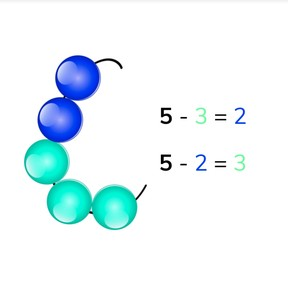Subtraction to 10 using related problems

# Subtraction to 10 using related problems

No account needed.8,000 schools use Gynzy92,000 teachers use Gynzy1,600,000 students use Gynzy

## General

Students learn to subtract numbers to 10 by using related problems.

1.OA.C.5
1.OA.C.6
1.OA.A

## Introduction

Start by decomposing the given numbers to complete their number bonds with visual support of beads. Count the number of red beads, white beads, and note the total. Students can also use blocks. You may choose to also do a few number decompositions verbally, asking students to name number bonds of numbers that you say aloud. Example: I can decompose the number 5 into 4 and ...". Then take a box with 10 marbles (or any other object). Say: "I have 6 marbles in my hand. I put 2 into the box. How many do I have left in my hand?". Then swap the amount set into the box and the difference. Set the 2 in your hand in the box and take the 4 from the box into your hand. Discover together with the students that you still have 6 in your hand, even though you now have 4 in your hand and 2 in the box. You can write the subtraction problems that represent this on the interactive whiteboard. Repeat this several times, starting with different number totals.

## Instruction

Using an image of beads on the interactive whiteboard, count the amount of beads on the string, the amount of beads in each color, and determine which subtraction problems can be made with this image. In this case you have 8 beads total, 5 orange and 3 blue beads. You can make the subtraction problems 8 - 5 = 3 and 8 - 3 = 5. If you know the difference of the first problem, you know the difference of the second problem as well, because the second number (subtrahend) and difference switch places. Practice with different amounts. Point at the beads when you first subtract them, and then point out that the number can swap places with the difference in the second subtraction problem. Be sure to stress that the first number (minuend), the total number, never moves from its place and is not changed at all. Ask students if they think the subtraction problem is made easier by swapping the second numbers (subtrahends) and differences. Some students like 5 - 1 = 4 than 5 - 4 = 1. Next show students the decomposition of 6 and the accompanying subtraction problems. Practice different number bond pair subtraction with your students. After practicing swapping the second number (subtrahend) and difference, tell students that they won't always have visual support for their subtraction problems. Show them a subtraction problem without visual support. Show them that they can still swap the second number (subtrahend) and difference and practice this with a few subtraction problems.

Check that students understand subtraction with numbers to ten using substitution by asking the following questions:
- You can decompose the number 8 into 2 and ...?
- 7 - 5 = 2. What is 7 -2?

## Quiz

Students first do subtraction problems with visual support, then with a visual rekenrek, and finally are given problems without visual support. They are asked to fill in the correct answer.

## Closing

Discuss with students that it is important to be able to do this, so you can calculate easier and faster. To close, finish with a few subtraction exercises. Have students check their work with their partner/seat-mate and discuss how they got their answer before discussing the answers as a class.

## Teaching tips

Students who have difficulty can be supported by the use of manipulatives like MAB blocks or by use of the rekenrek. Have them decompose a number like 8 into 3 and 5. Have them write the total number and then see if it makes a difference to write 8 - 3 or 8 - 5.

## Instruction materials

box, marbles (or other object)
optional: MAB-blocks, manipulatives, or rekenrek

### The online teaching platform for interactive whiteboards and displays in schools

• Save time building lessons

• Manage the classroom more efficiently

• Increase student engagement